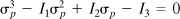## Appendix B Solution of the Stress Cubic Equation

### B.1. Principal Stresses

There are many methods in common usage for solving a cubic equation. A simple approach for dealing with Eq. (1.28) is to find one root, say σ1, by plotting it (σ as abscissa) or by trial and error. The cubic equation is then factored by dividing by (σp – σ1) to arrive at a quadratic equation. The remaining roots can be obtained by applying the familiar general solution of a quadratic equation. This process requires considerable time and algebraic work, however.

What follows is a practical approach for determining the roots of stress cubic equation (1.28):

(a)where

Get Advanced Strength and Applied Elasticity, Fourth Edition now with the O’Reilly learning platform.

O’Reilly members experience live online training, plus books, videos, and digital content from nearly 200 publishers.